• 采用窗函数法设计理想低通，高通滤波器，参考北京交通大学陈后金主编的【数字信号处理】5.2节 窗函数法设计线性相位FIR数字滤波器P164，和P188。 设计步骤如下： 1） 确定滤波器类型，不同的FIR类型可设计不同类型...
采用窗函数法设计理想低通，高通滤波器，参考北京交通大学陈后金主编的【数字信号处理】5.2节 窗函数法设计线性相位FIR数字滤波器P164，和P188。

设计步骤如下：

1） 确定滤波器类型，不同的FIR类型可设计不同类型的滤波器，I型可设计LP(低通滤波器)，HP(高通滤波器)，BP(带通滤波器)，BS(带阻滤波器)。

Fir I型

Fir II型

Fir III型

Fir IV型

LP，HP，BP，BS

LP，BP

BP

HP，BP，BS

2） 确定设计的滤波器的参数

Eg：若要设计一个低通滤波器，fp=20，fs=30；Ap=1，As=40，则3db截频Wc = 2*pi*（fs-fp）/Fs；Fs为采样频率。

当选定某一窗函数时，衰耗Ap和As就已经确定，凯撒窗除外。Ap和As的计算方法可参看另外一篇博客： https://www.cnblogs.com/xhslovecx/p/10118570.html

3） 确定窗函数

窗的类型

主瓣宽度

近似过渡带宽度

δp，δs

Ap（dB）

As（dB）

矩形窗

4pi/N

1.8pi/N

0.09

0.82

21

Hann

8pi/N

6.2pi/N

0.0064

0.056

44

Hamming

8pi/N

7pi/N

0.0022

0.019

53

Blackman

12pi/N

11.4pi/N

0.0002

0.0017

74

Kaiser

可调窗，需要确定 β值

50<A ,            β = 0.1102（A-8.7）；

21<=A<=50,   β=0.5842(A-21)^0.4 + 0.07886(A-21)；

A<21,             β = 0；

4) 确定滤波器的阶数M，首先确定滤波器的长度N。对于除凯撒窗以外的窗函数，N值由以下公式确定：

N>=(窗函数近似过渡带宽度)/（Wp-Ws）

Fir I型

Fir II型

Fir III型

Fir IV型

脉冲响应h[k]为偶对称

h[k]为偶对称

h[k]为奇对称

h[k]为奇对称

窗函数长度：N=mod(N+1,2)+N

N=mod(N,2)+N

N=mod(N+1,2)+N

N=mod(N,2)+N

阶数M=N-1为偶数

M=N-1为奇数

M=N-1为偶数

M=N-1为奇数

若采用Kaiser窗，则

M≈ （A-7.95）÷ 2.285*|Wp-Ws|，A>21。其中，A = -20lg（min（δp，δs））

5) 理想滤波器脉冲信号如下：

hd = (Wc/pi)*sinc(Wc*(k-0.5*M)/pi);%低通

hd = -(Wc/pi)*sinc(Wc*(k-0.5*M)/pi);%高通

6) 加窗：

W = hanning(N);  W = hamming(N);  W = blackman(N);    N = M+1;

W = kaiser(N,beta);

7) 截断

h = hd.*W;

8）滤波

sigFiltered = filter(h,,signal);

matlab代码实现详见：FIR I 型 滤波器设计
展开全文• [Matlab]FIR滤波器设计：(基本窗函数FIR滤波器设计) ​ IIR滤波器主要设计方法先设计一个模拟低通滤波器，然后把它转化为形式上的数字滤波器。...FIR滤波器的设计方法主要有窗函数法、频率采样法、切比雪夫逼近法等...
[Matlab]FIR滤波器设计：(基本窗函数FIR滤波器设计)
​	IIR滤波器主要设计方法先设计一个模拟低通滤波器，然后把它转化为形式上的数字滤波器。但对于FIR滤波器来说，设计方法的关键要求之一就是保证线性相位条件。而IIR滤波器的设计方法中只对幅值特性进行设计，因此无法保证相位。所以FIR滤波器的设计需要采用完全不同的方法。FIR滤波器的设计方法主要有窗函数法、频率采样法、切比雪夫逼近法等。
​	由于理想滤波器在边界频率处不连续，故时域信号$h_d(n)$一定是无限时宽的，也是非因果的。所以理想低通滤波器是无法实现的。如果实现一个具有理想线性相位特性的滤波器，其幅值特性只能采用逼近理想幅频特性的方法实现。如果对时域信号$h_d(n)$进行截取，并保证截取过程中序列保持对称，而且截取长度为N,则对称点为$α=\frac{1}{2}*(N-1)$。截取后序列为$h(n)$，侧$h(n)$可用下式子表示：
$h(n) = h_d(n)*w(n)$
式中，$w(n)$为截取函数，又称为穿函数。如果窗函数为矩形序列，则称之为矩形窗。窗函数有多种形式，为保证加窗后系统的线性相位特性，必须保证加窗后序列关于$α=\frac{1}{2}*(N-1)$点对称。常用的窗函数有矩形窗、汉宁窗、汉明窗、布莱克曼窗、凯塞窗。窗函数设计法的基本思想是用一个长度为N的序列$h(n)$代替$h_d(n)$作为实际设计的滤波器的单位脉冲响应。这种设计法成为窗函数设计法。显然在保证$h(n)$对称性的前提下，窗函数长度N越长，则$h(n)$越接近$h_d(n)$。但是误差是肯定存在的，这种误差成为截断误差。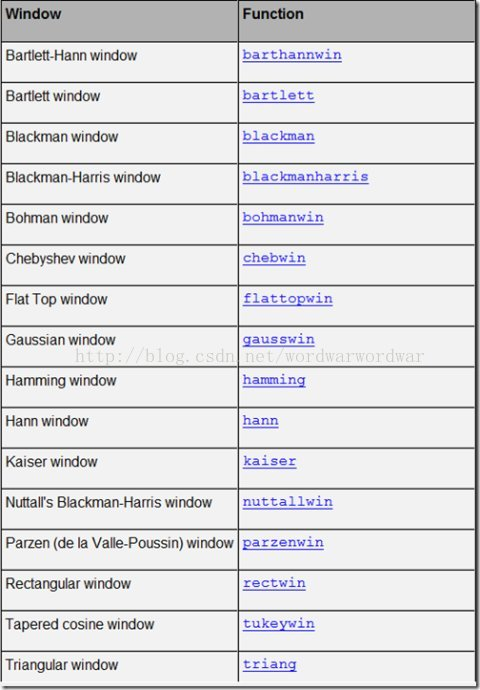​	要确定如何设计一个FIR滤波器，首先得对加窗后的理想滤波器特性变化进行分析，并研究减少由截断引起的误差的途径，从而提出更好的滤波器设计方案。对于调整窗口长度可以有效地控制过渡带的宽度，但减少带内波动以及加大阻带衰减没有作用。所以必须挑选最为合适的窗函数对理想滤波器进行截取。下面简单介绍几种窗函数。一个实际的滤波器的单位脉冲响应可表示为：
$h(n) = h_d(n)*w(n)$
几种常见窗函数（只给出了窗函数的定义和幅度特性）：
$W(e^{j*w})=W(w)(e^{-jαw})$
矩形窗FIR滤波器设计:
矩形窗的窗函数为：
$w_R(n)=R_N(n)$
幅度函数为：
$R_N(w) = \frac{sin(wN/2)}{sin(w/2)}$
它的主瓣宽度为$4\pi/N$,第一瓣比主瓣地13dB.
在Matlab中，实现矩形窗的函数为boxcar和recttwin ,其调用格式如下：
w=boxcar(N);
w=recttwin(N);
%%%显示窗函数的GUI工具
n = 60;
w = rectwin(n);
wvtool(w)%显示窗函数的GUI工具
%还提供了显示窗函数的GUI工具，如wvtool可以显示用来显示窗的形状和频域图形，wintool可以打开窗设计和分析工具，如运行
wvtool(hamming(64),hann(64),gausswin(64))
%%可以对比汉明窗、汉宁窗和高斯窗

其中，N是窗函数的长度，返回值w是一个N阶的向量，它的元素有窗函数的值组成。其中w=boxcar(N)等价于w=ones(N,1)。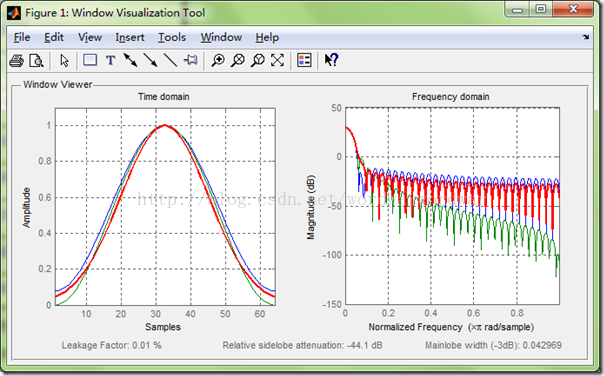案例分析：
利用矩形窗设计FIR带阻滤波器，主要参数如下:
给定抽样频率为$Ωs=2*pi*1.5*10^4(rad/sec)$,
通带截至频率为$Ωp1=2*pi*0.75*10^3(rad/sec),Ωp2=2*pi*6*10^3(rad/sec)$
阻带截至频率为$Ωst1=2*pi*2.25*10^3(rad/sec),Ωst2=2*pi*1.5*10^3(rad/sec)$
阻带衰减${\delta}_2 >=50dB$。
%%%%调用子程序1：
function hd=ideal_bs(Wcl,Wch,m);
alpha=(m-1)/2;
n=[0:1:(m-1)];
m=n-alpha+eps;
hd=[sin(m*pi)+sin(Wcl*m)-sin(Wch*m)]./(pi*m)
%%%%调用子程序2：
function[db,mag,pha,w]=freqz_m2(b,a)
[H,w]=freqz(b,a,1000,'whole');
H=(H(1:1:501))'; w=(w(1:1:501))';
mag=abs(H);
db=20*log10((mag+eps)/max(mag));
pha=angle(H);
%%%%运行MATLAB源代码如下：
clear all;
Wph=3*pi*6.25/15;%通带频率
Wpl=3*pi/15;
Wsl=3*pi*2.5/15;%阻带频率
Wsh=3*pi*4.75/15;
tr_width=min((Wsl-Wpl),(Wph-Wsh));%%过渡带带宽
%过渡带宽度
N=ceil(4*pi/tr_width);					%滤波器长度
n=0:1:N-1;
Wcl=(Wsl+Wpl)/2;						%理想滤波器的截止频率
Wch=(Wsh+Wph)/2;
hd=ideal_bs(Wcl,Wch,N);				%理想滤波器的单位冲击响应
w_ham=(boxcar(N))';
string=['矩形窗','N=',num2str(N)];
h=hd.*w_ham;						%截取取得实际的单位脉冲响应
[db,mag,pha,w]=freqz_m2(h,);
%计算实际滤波器的幅度响应
delta_w=2*pi/1000;
subplot(241);
stem(n,hd);
title('理想脉冲响应hd(n)')
axis([-1,N,-0.5,0.8]);
xlabel('n');ylabel('hd(n)');
grid on
subplot(242);
stem(n,w_ham);
axis([-1,N,0,1.1]);
xlabel('n');ylabel('w(n)');
text(1.5,1.3,string);
grid on
subplot(243);
stem(n,h);title('实际脉冲响应h(n)');
axis([0,N,-1.4,1.4]);
xlabel('n');ylabel('h(n)');
grid on
subplot(244);
plot(w,pha);title('相频特性');
axis([0,3.15,-4,4]);
xlabel('频率（rad）');ylabel('相位（Φ）');
grid on
subplot(245);
plot(w/pi,db);title('幅度特性（dB）');
axis([0,1,-80,10]);
xlabel('频率（pi）');ylabel('分贝数');
grid on
subplot(246);
plot(w,mag);title('频率特性')
axis([0,3,0,2]);
xlabel('频率（rad）');ylabel('幅值');
grid on
fs=15000;
t=(0:100)/fs;
x=cos(2*pi*t*750)+cos(2*pi*t*3000)+cos(2*pi*t*6100);
q=filter(h,1,x);
[a,f1]=freqz(x);
f1=f1/pi*fs/2;
[b,f2]=freqz(q);
f2=f2/pi*fs/2;
subplot(247);
plot(f1,abs(a));
title('输入波形频谱图');
xlabel('频率');ylabel('幅度')
grid on
subplot(248);
plot(f2,abs(b));
title('输出波形频谱图');
xlabel('频率');ylabel('幅度')
grid on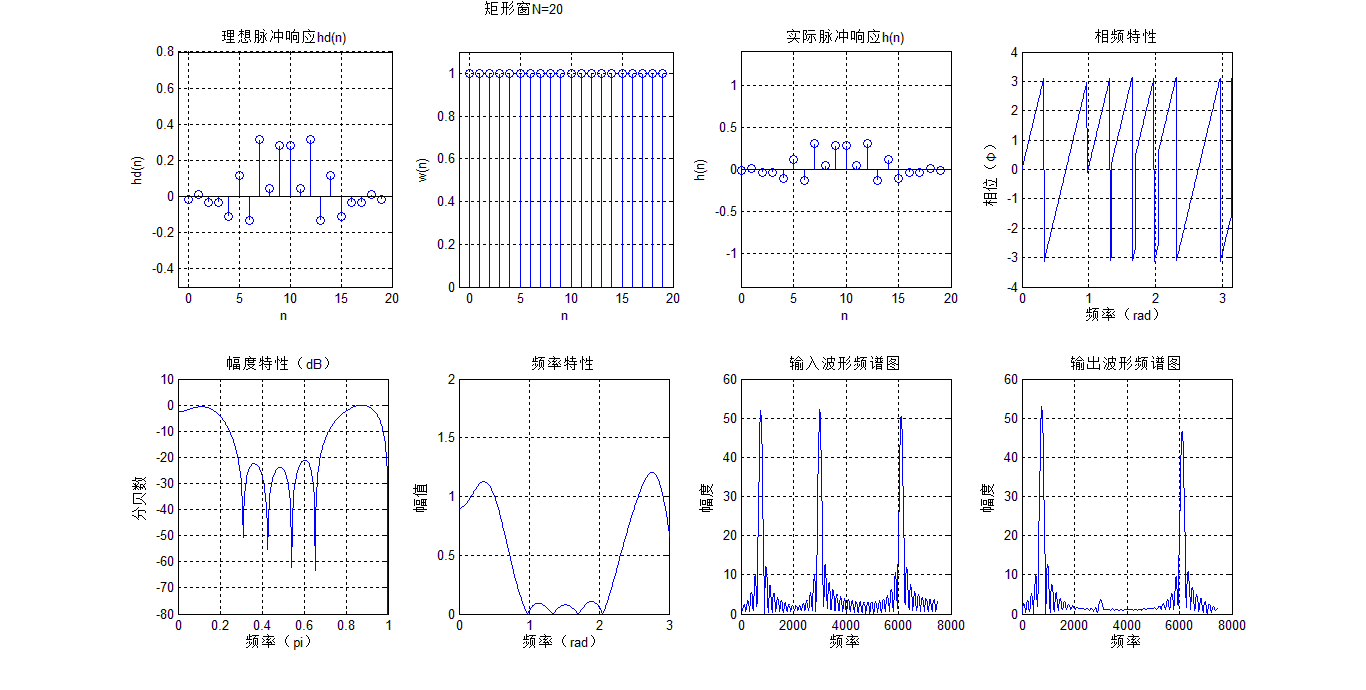汉宁窗FIR滤波器设计:
汉宁窗（hanning window）又称升余弦窗，窗函数为：
$w_{Hn}(n) = 0.5[1-cos(\frac{2\pi*n}{N-1})]*R_N(n)$
幅值函数为：
$W_{Hn}(w) = 0.5W_R(w) + 0.25[W_R(w-\frac{2\pi}{N-1})+W_R(w+\frac{2\pi}{N-1})]$
汉宁窗幅度函数由3部分相加而成，其结果是使主瓣集中了更多能量，而旁瓣3部分相加时相互抵消而变小，其代价是主瓣宽度增加到$8\pi/N$。第一瓣比主瓣低31dB,阻带衰减加大。
在Matlab中，实现汉宁窗的函数为hanning和barthannwin ,其调用格式如下：
w=hanning(N)
w=barthannwin(N)

案例1：绘制50个点的汉宁窗。
N=49;n=1:N;
wdhn=hanning(N);
figure(3);
stem(n,wdhn,'.');
grid on
axis([0,N,0,1.1]);
title('50点汉宁窗');
ylabel('W(n)');
xlabel('n');
title('50点汉宁窗');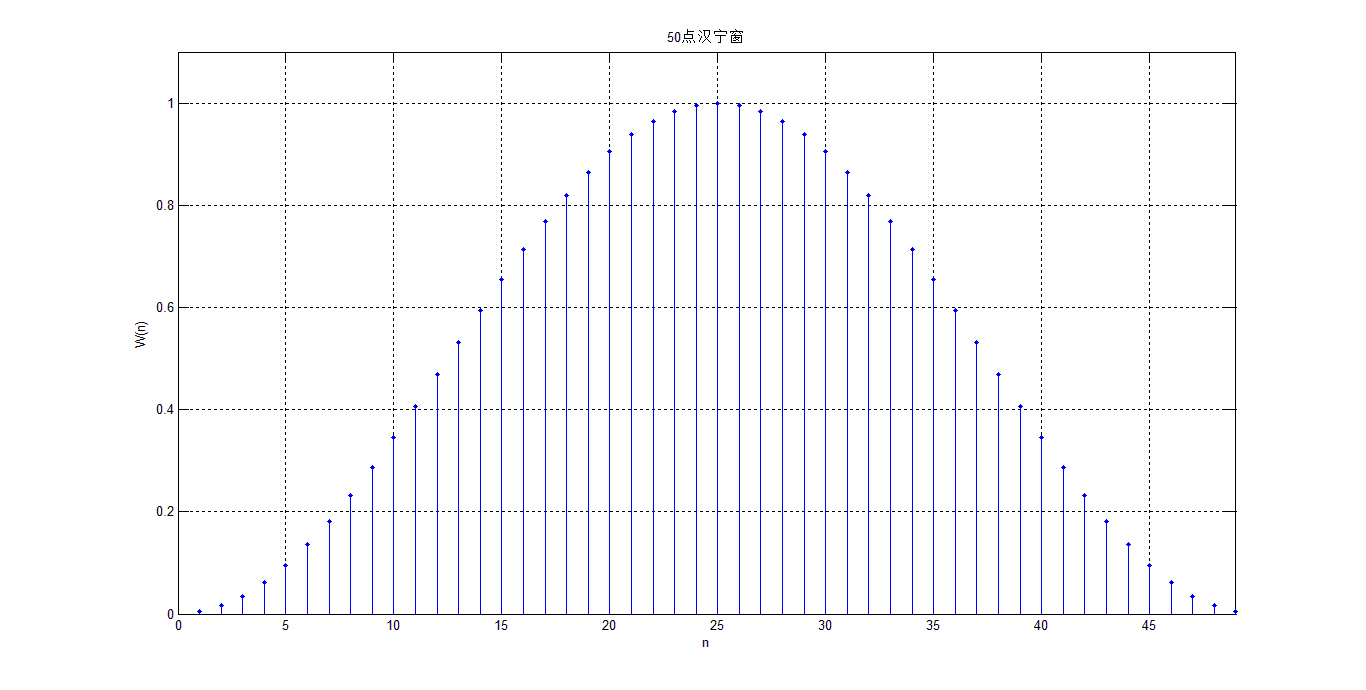案例2：已知连续信号为$x(t)=cos(2{\pi}f_1t) +0.15cos(2{\pi}f_2t)$，其中$f_1=100Hz,f_2=150Hz$。若抽样频率$f_sam=600Hz$d对信号进行抽样，利用不同宽度N的矩形截断该序列，N取40，观察不同的窗对普分析结果的影响。
N=40;
L=512;
f1=100;f2=150;fs=600;
ws=2*pi*fs;
t=(0:N-1)*(1/fs);
x=cos(2*pi*f1*t)+0.25*sin (2*pi*f2*t);
wh=boxcar(N)';
x=x.*wh;
subplot(221);stem(t,x);
title('加矩形窗时域图');
xlabel('n');ylabel('h(n)')
grid on
W=fft(x,L);
f=((-L/2:L/2-1)*(2*pi/L)*fs)/(2*pi);
subplot(222);
plot(f,abs(fftshift(W)))
title('加矩形窗频域图');
xlabel('频率');ylabel('幅度')
grid on
x=cos(2*pi*f1*t)+0.15*cos(2*pi*f2*t);
wh=hanning(N)';
x=x.*wh;
subplot(223);stem(t,x);
title('加汉宁窗时域图');
xlabel('n');ylabel('h(n)')
grid on
W=fft(x,L);
f=((-L/2:L/2-1)*(2*pi/L)*fs)/(2*pi);
subplot(224);
plot(f,abs(fftshift(W)))
title('加汉宁窗频域图');
xlabel('频率');ylabel('幅度')
grid on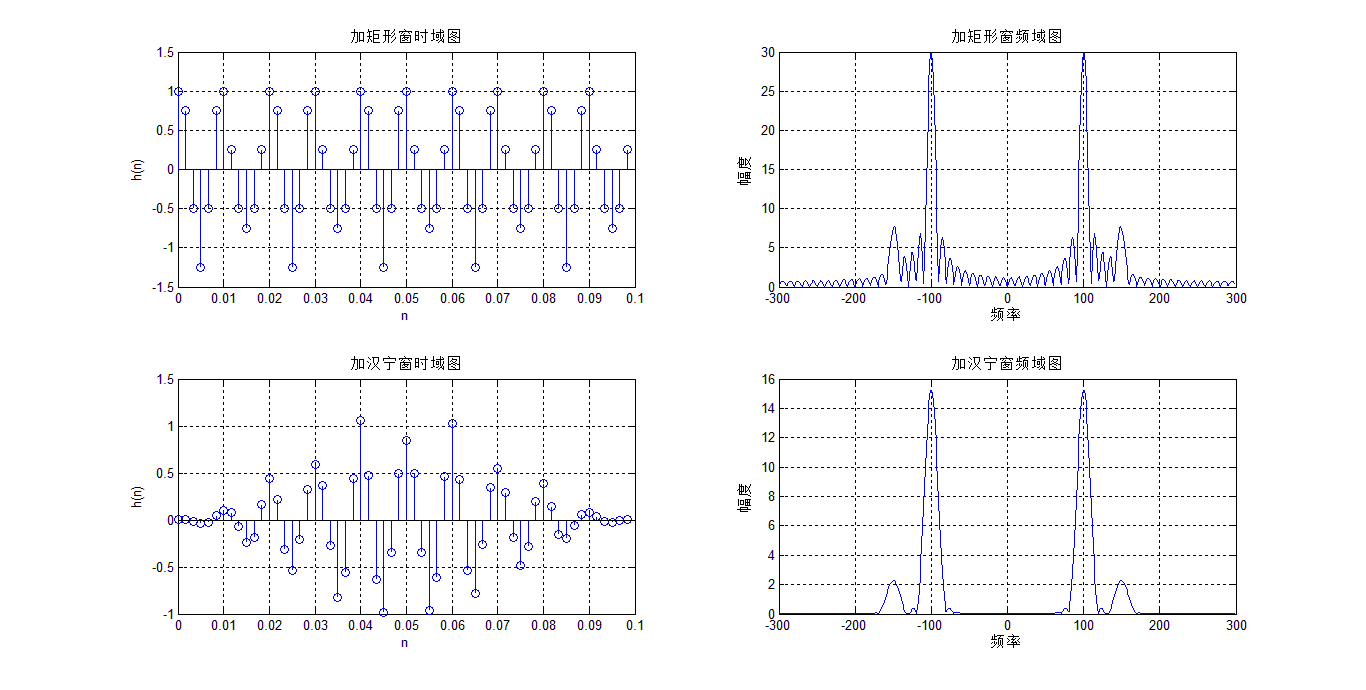用汉宁窗对谐波信号进行分析：
clear;
% 原始数据：直流:0V; 基波：49.5Hz,100V,10deg; HR2:0.5V,40deg;
hr0=0;f1=50.1;
hr(1)=25*sqrt(2);deg(1)=10;
hr(2)=0;deg(2)=0;
hr(3)=1.755*sqrt(2);deg(3)=40;
hr(4)=0;deg(4)=0;
hr(5)=0.885*sqrt(2);deg(5)=70;
hr(6)=0;deg(6)=0;
hr(7)=1.125;deg(7)=110;
M=7;f=[1:M]*f1;							%设定频率
% 采样
fs=10000;
N=2048;									% 约10个周期
T=1/fs;
n=[0:N-1];t=n*T;
x=zeros(size(t));
for k=1:M
x=x+hr(k)*cos(2*pi*f(k)*t+deg(k)*pi/180);
end
%分析：
w=0.5-0.5*cos(2*pi*n/N);
Xk=fft(x.*w);
amp=abs(Xk(1:N/2))/N*2;						%幅频
pha=angle(Xk(1:N/2))/pi*180;					%相频
for k=1:N/2
if(amp(k)<0.01) pha(k)=0;  %当谐波<10mV时，其相位=0
end
if(pha(k)<0) pha(k)=pha(k)+360;%调整到0-360度
end
end
fmin=fs/N;
xaxis=fmin*n(1:N/2);
%横坐标为Hz
kx=round([1:M]*50/fmin);
%各次谐波对应的下标(从0开始)
for m=1:M
km(m)=searchpeaks(amp,kx(m)+1);			%km为谱峰(从1开始)
if(amp(km(m)+1)<amp(km(m)-1))
km(m)=km(m)-1;
end
beta(m)=amp(km(m)+1)./amp(km(m));
delta(m)=(2*beta(m)-1)./(1+beta(m));
end
fx=(km-1+delta)*fmin;							%估计频率
hrx=amp(km)*2.*pi.*delta.*(1-delta.*delta)./sin(pi*delta);
degx=pha(km)-delta.*180/N*(N-1);				%估计相位
degx=mod(degx,360);							%调整到0-360度
efx=(fx-f)./f*100;								%频率误差
ehr=(hrx-hr)./hr*100;							%幅度误差
edeg=(degx-deg);								%相位误差
% 结果输出：
subplot(2,2,1);
%画出采样序列
plot(t,x);
hold on;
plot(t,x.*w,'r');
%加窗波形
hold off;
xlabel('x(k)');
title('原信号和加窗信号 ');
subplot(2,2,2);
%画出FFT分析结果
stem(xaxis,amp,'.r');
xlabel('频率');
title('幅频结果');
subplot(2,2,4);
stem(xaxis,pha,'.r');
xlabel('角频率');
title('相频结果');
subplot(2,2,3);
stem(ehr);
title('幅度误差(%)');
%文本输出
fid=fopen('result.txt','w');
fprintf(fid,'原始数据：f1=%6.1fHz, N=%.f,  fs=%.f \r\n\r\n',f1,N,fs);
fprintf(fid,'谐波次数      1      2      3      4      5      6     7\r\n');
fprintf(fid,'设定频率 %6.3f %6.3f %6.3f %6.3f %6.3f %6.3f %6.3f\r\n',f);
fprintf(fid,'估计频率 %6.3f %6.3f %6.3f %6.3f %6.3f %6.3f %6.3f\r\n',fx);
fprintf(fid,'误差(%%)  %6.3f %6.3f %6.3f %6.3f %6.3f %6.3f %6.3f\r\n\r\n',efx);
fprintf(fid,'设定幅值 %6.3f %6.3f %6.3f %6.3f %6.3f %6.3f %6.3f\r\n',hr);
fprintf(fid,'估计幅值 %6.3f %6.3f %6.3f %6.3f %6.3f %6.3f %6.3f\r\n',hrx);
fprintf(fid,'误差(%%)  %6.3f %6.3f %6.3f %6.3f %6.3f %6.3f %6.3f\r\n\r\n',ehr);
fprintf(fid,'设定相位 %6.2f %6.2f %6.2f %6.2f %6.2f %6.2f %6.2f\r\n',deg);
fprintf(fid,'估计相位 %6.2f %6.2f %6.2f %6.2f %6.2f %6.2f %6.2f\r\n',degx);
fprintf(fid,'误差(度)  %6.2f %6.2f %6.2f %6.2f %6.2f %6.2f %6.2f\r\n\r\n',edeg);
%其他数据
fprintf(fid,'谱峰位置理论值：\r\n %6.4f %6.4f %6.4f %6.4f %6.4f %6.4f %6.4f\r\n',[1:M]*f1/fmin);
fprintf(fid,'谱峰位置估计值：\r\n %6.4f %6.4f %6.4f %6.4f %6.4f %6.4f %6.4f\r\n',km-1+delta);
fprintf(fid,'误差（%%）\r\n %6.4f %6.4f %6.4f %6.4f %6.4f %6.4f %6.4f\r\n',((km-1+delta)-[1:M]*f1/fmin)./([1:M]*f1/fmin)*100);
fprintf(fid,'delta     ：\r\n %6.4f %6.4f %6.4f %6.4f %6.4f %6.4f %6.4f\r\n',delta);
fclose(fid);

%运行过程中的调用子程序：
function index1=searchpeaks(x,index)
%在数组中寻找最大值对应的下标
%x为数组，index 为给定的下标(index不能取最前或最后2个下标)，在前后2个数中（共5个数）查找最大值和紧邻的次最大值
% indexmax 返回两个谱峰位置中的前一个谱峰对应的下标
index1=index-2;
for k=-1:2
if(x(index+k)>x(index1))
index1=index+k;
end
end
if x(index1-1)>x(index1+1)
index1=index1-1;
end


#result.txt输出结果
原始数据：f1=  50.1Hz, N=2048,  fs=10000

谐波次数      1      2      3      4      5      6     7
设定频率 50.100 100.200 150.300 200.400 250.500 300.600 350.700
估计频率 50.100 78.819 150.302 181.252 250.499 279.138 350.701
误差(%)  -0.000 -21.338  0.001 -9.555 -0.000 -7.140  0.000

设定幅值 35.355  0.000  2.482  0.000  1.252  0.000  1.125
估计幅值 35.356  0.046  2.482  0.002  1.252  0.002  1.125
误差(%)   0.001    Inf  0.009    Inf  0.004    Inf  0.003

设定相位  10.00   0.00  40.00   0.00  70.00   0.00 110.00
估计相位  10.03  31.67  39.97 338.35  70.06 329.86 110.05
误差(度)    0.03  31.67  -0.03 338.35   0.06 329.86   0.05

谱峰位置理论值：
10.2605 20.5210 30.7814 41.0419 51.3024 61.5629 71.8234
谱峰位置估计值：
10.2605 16.1421 30.7818 37.1203 51.3022 57.1675 71.8235
误差（%）
-0.0002 -21.3385 0.0012 -9.5551 -0.0004 -7.1396 0.0002
delta     ：
0.2605 0.1421 0.7818 0.1203 0.3022 0.1675 0.8235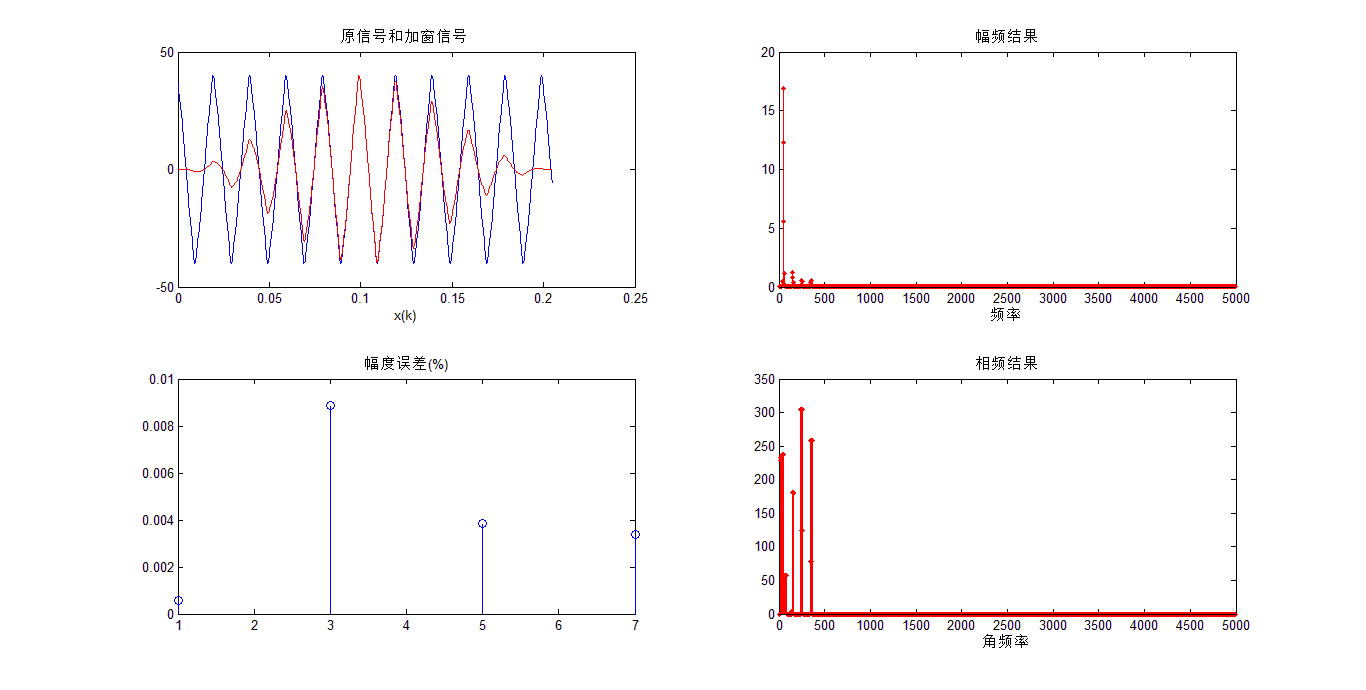汉明窗FIR滤波器设计:
汉宁窗（hanming window）又称改进升余弦窗，窗函数为：
$w_{Hn}(n) = [0.54-0.46*cos(\frac{2\pi*n}{N-1})]*R_N(n)$
幅值函数为：
$W_{Hn}(w) = 0.54W_R(w) + 0.23[W_R(w-\frac{2\pi}{N-1})+0.23W_R(w+\frac{2\pi}{N-1})]$
汉明窗主瓣宽度与汉宁窗相同，$8{\pi}/N，99.96\%$的能量集中在主瓣，第一瓣比主瓣低41dB。
在Matlab中，实现汉宁窗的函数为hanming ,其调用格式如下：
w=hanming(N)

案例分析1：设计一个汉明窗低通滤波器：
%语音信号设计一个汉明窗低通滤波器：
[x,FS,bits]=wavread('C:\Windows\Media\Windows Ringout');
x=x(:,1);
figure(1);
subplot(211);plot(x);
title('语音信号时域波形图')
xlabel('n');ylabel('h(n)')
grid on
y=fft(x,1000);
f=(FS/1000)*[1:1000];
subplot(212);
plot(f(1:300),abs(y(1:300)));
title('语音信号频谱图');
xlabel('频率');ylabel('幅度')
grid on
%产生噪声信号并加到语音信号
t=0:length(x)-1;
zs0=0.05*cos(2*pi*10000*t/1024);
zs=[zeros(0,20000),zs0];
figure(2);
subplot(211)
plot(zs)
title('噪声信号波形');
xlabel('n');ylabel('h(n)')
grid on
zs1=fft(zs,1200);
subplot(212)
plot(f(1:600),abs(zs1(1:600)));
title('噪声信号频谱');
xlabel('频率');ylabel('幅度')
grid on
x1=x+zs';
%sound(x1,FS,bits);
y1=fft(x1,1200);
figure(3);
subplot(211);plot(x1);
title('加入噪声后的信号波形');
xlabel('n');ylabel('h(n)')
grid on
subplot(212);
plot(f(1:600),abs(y1(1:600)));
title('加入噪声后的信号频谱');
xlabel('频率');ylabel('幅度')
grid on
%滤波
fp=7500;
fc=8500;
wp=2*pi*fp/FS;
ws=2*pi*fc/FS;
Bt=ws-wp;
N0=ceil(6.2*pi/Bt);
N=N0+mod(N0+1,2);
wc=(wp+ws)/2/pi;
hn=fir1(N-1,wc,hamming(N));
X=conv(hn,x);
X1=fft(X,1200);
figure(4);
subplot(211);
plot(X);
title('滤波后的信号波形');
xlabel('n');ylabel('h(n)')
grid on
subplot(212);
plot(f(1:600),abs(X1(1:600)));
title('滤波后的信号频谱')
xlabel('频率');ylabel('幅度')
grid on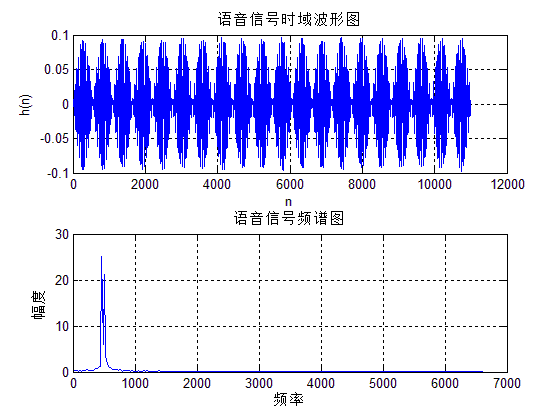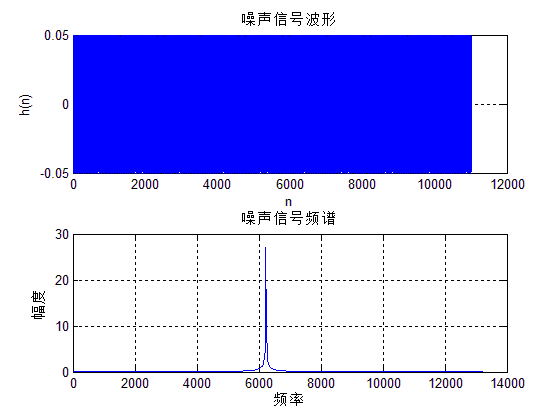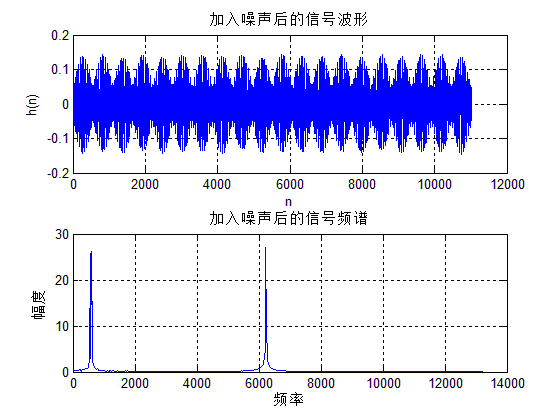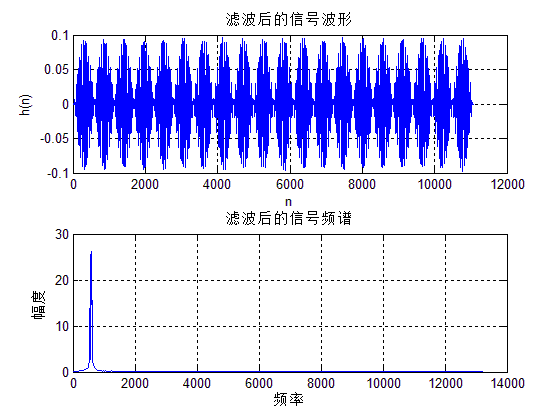案例分析2：已知连续信号为$x_a(t)=cos(100{\pi}t) +sin(100{\pi}t)+cos(50{\pi}t)$，用DFT分析其中$x_a(t)$的频谱结构，选择不同的截取长度$T_p$。观察存在的截断效应，试用加窗的方法减少谱间干扰。
clear;close all
fs=400;T=1/fs;						%采样频率和采样间隔
Tp=0.04;N=Tp*fs;					%采样点数N
N1=[N,4*N,8*N];					%设定三种截取长度
for m=1:3
n=1:N1(m);
xn=cos(100*pi*n*T)+ sin(200*pi*n*T)+ cos(50*pi*n*T);
Xk=fft(xn,4096);
fk=[0:4095]/4096/T;
subplot(3,2,2*m-1);plot(fk,abs(Xk)/max(abs(Xk)));
if m==1 title('矩形窗截取');
end
end
%hamming窗截断
for m=1:3
n=1:N1(m);
wn=hamming(N1(m));
xn=cos(200*pi*n*T)+ sin(100*pi*n*T)+ cos(50*pi*n*T).*wn';
Xk=fft(xn,4096);
fk=[0:4095]/4096/T;
subplot(3,2,2*m);plot(fk,abs(Xk)/max(abs(Xk)));
if m==1 title('hamming窗截取');
end
end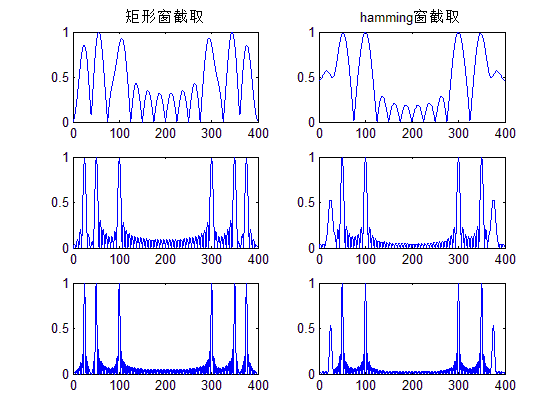比较矩形窗与汉明窗的普分析结果可见，矩形窗比用汉明窗分辨率高（泄露小），但是谱间干扰大。因此汉明窗是以牺牲分辨率来换取谱间干扰降低。
布莱克曼FIR滤波器设计:
布莱克曼（Blackman window）的窗函数为：
$w_{Bl}(n) = [0.42-0.5*cos(\frac{2\pi*n}{N-1})+0.08*cos(\frac{4\pi*n}{N-1})]*R_N(n)$
幅值函数为：
$W_{Hn}(w) = 0.42W_R(w) + 0.25[W_R(w-\frac{2\pi}{N-1})+W_R(w+\frac{2\pi}{N-1})]$
$+0.04[W_R(w-\frac{4\pi}{N-1}+W_R(w+\frac{4\pi}{N-1})]$
布莱克曼窗幅度函数由5部分相加而成，5部分相加的结果使得旁瓣得到进一步抵消，阻带衰减加大而过渡带加大到$12{\pi}/N$。
在Matlab中，实现布莱克曼窗的函数为blackman ,其调用格式如下：
w=blackman(N);

:案例：用窗函数法设计数字带通滤波器。下阻带边缘：$W_{s1}=0.3{\pi}，A_s=65dB$，下通带边缘:$W_{p1}=0.4{\pi}，R_p=1dB$,上通带边缘：$W_{p2}=0.6{\pi}，R_p=1dB$，上阻带边缘:$W_{s2}=0.7{\pi}，R_p=65dB$。根据窗函数最小阻带衰减的特性，以及参照窗函数的基本参数表，选择布莱克曼窗可以达到75dB的最小阻带衰减，其过渡带为$11\pi/N$。
clear all;
wp1=0.4*pi;
wp2=0.6*pi;
ws1=0.3*pi;
ws2=0.7*pi;
As=65;
tr_width=min((wp1-ws1),(ws2-wp2)); 					%过渡带宽度
M=ceil(11*pi/tr_width)+1							%滤波器长度
n=[0:1:M-1];
wc1=(ws1+wp1)/2;									%理想带通滤波器的下截止频率
wc2=(ws2+wp2)/2;									%理想带通滤波器的上截止频率
hd=ideal_lp(wc2,M)-ideal_lp(wc1,M);
w_bla=(blackman(M))';								%布莱克曼窗
h=hd.*w_bla;
%截取得到实际的单位脉冲响应
[db,mag,pha,grd,w]=freqz_m(h,);
%计算实际滤波器的幅度响应
delta_w=2*pi/1000;
Rp=-min(db(wp1/delta_w+1:1:wp2/delta_w))
%实际通带纹波
As=-round(max(db(ws2/delta_w+1:1:501)))
As=75
subplot(2,2,1);
stem(n,hd);
title('理想单位脉冲响应hd(n)')
axis([0 M-1 -0.4 0.5]);
xlabel('n');
ylabel('hd(n)')
grid on;
subplot(2,2,2);
stem(n,w_bla);
title('布莱克曼窗w(n)')
axis([0 M-1 0 1.1]);
xlabel('n');
ylabel('w(n)')
grid on;
subplot(2,2,3);
stem(n,h);
title('实际单位脉冲响应hd(n)')
axis([0 M-1 -0.4 0.5]);
xlabel('n');
ylabel('h(n)')
grid on;
subplot(2,2,4);
plot(w/pi,db);
axis([0 1 -150 10]);
title('幅度响应(dB)');
grid on;
xlabel('频率单位:pi');
ylabel('分贝数')

%调用小程序设计1：
function [db,mag,pha,grd,w] = freqz_m(b,a);
% Modified version of freqz subroutine
% ------------------------------------
% [db,mag,pha,grd,w] = freqz_m(b,a);
%  db = Relative magnitude in dB computed over 0 to pi radians
% mag = absolute magnitude computed over 0 to pi radians
% pha = Phase response in radians over 0 to pi radians
% grd = Group delay over 0 to pi radians
%   w = 501 frequency samples between 0 to pi radians
%   b = numerator polynomial of H(z)   (for FIR: b=h)
%   a = denominator polynomial of H(z) (for FIR: a=)
%
[H,w] = freqz(b,a,1000,'whole');
H = (H(1:1:501))'; w = (w(1:1:501))';
mag = abs(H);
db = 20*log10((mag+eps)/max(mag));
pha = angle(H);
%  pha = unwrap(angle(H));
grd = grpdelay(b,a,w);
%  grd = diff(pha);
%  grd = [grd(1) grd];
%  grd = [0 grd(1:1:500); grd; grd(2:1:501) 0];
%  grd = median(grd)*500/pi;

%调用小程序设计2：
function hd=ideal_lp(wc,M);
%计算理想低通滤波器的脉冲响应
%[hd]=ideal_lp(wc,M)
%hd=理想脉冲响应0到M-1
%wc=截止频率
% M=理想滤波器的长度
alpha=(M-1)/2;
n=[0:1:(M-1)];
m=n-alpha+eps;
%加上一个很小的值eps避免除以0的错误情况出现
hd=sin(wc*m)./(pi*m);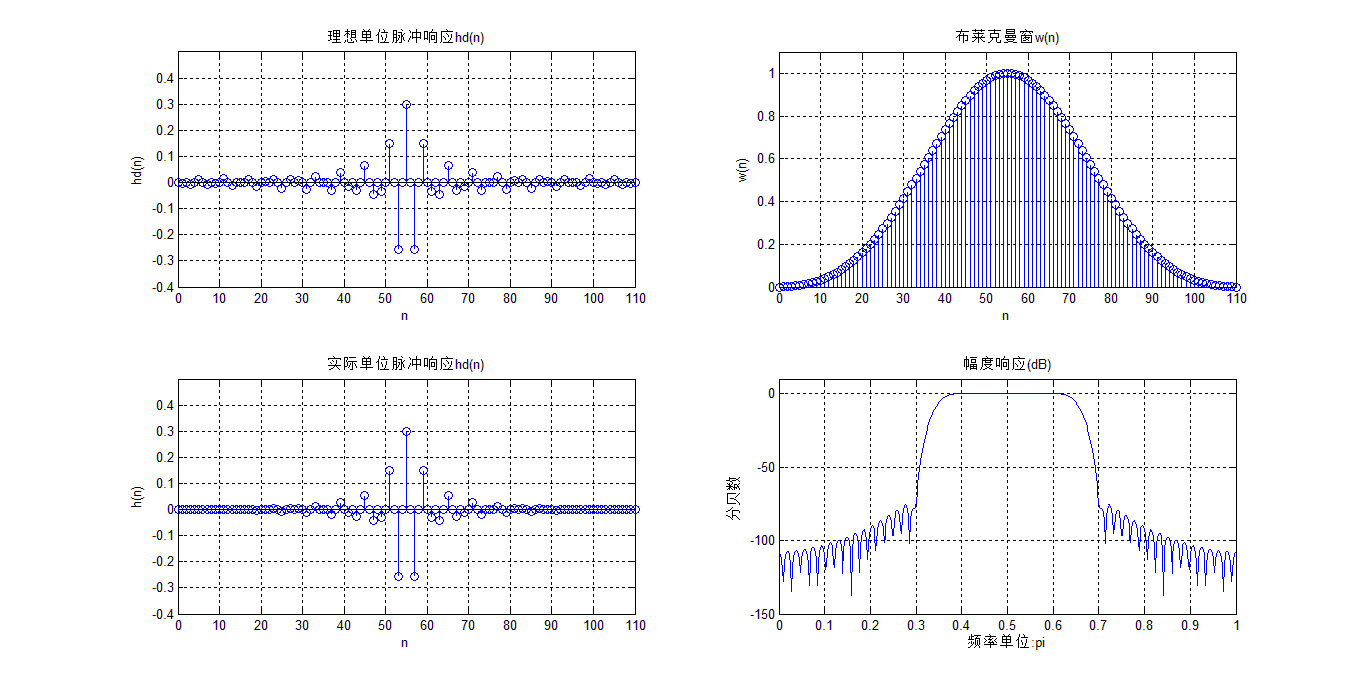凯塞窗FIR滤波器设计:
凯塞-贝塞窗（Kaiser-Basel window)的窗函数为：
$w_{k}(n) = \frac{I_0( β )}{I_0( \alpha )}*R_N(n)$
式中，$\beta= {\alpha}{\sqrt{(1-(\frac{2n}{N-1})-1)^2}}$。 $I_0( x )$是零阶第一类修正贝塞函数，可用下面级数计算：
$I_0( x ) = 1+\sum_{k=1}^{+ ∞}（\frac{1}{k!}({\frac{x}{2}})^{k}）^{2}$
​	$I_0( x )$q取15-25项就可以满足精度要求。通常$\alpha$用以控制窗的形状，$\alpha$加大，主瓣加宽，旁瓣减小，典型数据$4<\alpha<9$。当$\alpha=5.44$s时，窗函数接近汉明窗；当$\alpha=7.865$s时，窗函数接近于布莱克曼窗。其幅值函数为：
$W_{k}(w) =w_k(0) + 2\sum_{n=1}^{\frac{(N-1)}{2}}(w_k(n)cos(wn))$
在Matlab中，实现汉宁窗的函数为kaiser,其调用格式如下：
w=kaiser(N);
在Matlab中设计标准响应FIR滤波器可使用fir1函数。fir1函数以经典方法实现加窗性相位FIR滤波器的设计，它可以设计出标准的低通、高通、带通、带阻滤波器。fir1函数用法为：
b = fir1(n,Wn,‘ftype’,wimdow)
各个参数的含义如下：

b -滤波器系数，n-滤波器阶数。
Wn -截止频率，$0<=W_n<=1$,$W_n=1$对应于采样频率的一半。当设计带通和带阻滤波器时，$W_n =[W_1,W_2],W_1
ftype -当指定ftype时，可设计高通和带阻滤波器。ftype=hight时，设计高通FIR滤波器；ftype=stop时设计带阻FIR滤波器。低通和带通FIR滤波器无需输入ftype参数。
window–窗函数。窗函数的长度应等于FIR滤波器系数的个数，即阶数n+1。

案例分析：利用凯塞窗函数设计一个带通滤波器，上截止频率2500Hz,下截止频率1000Hz，过渡带宽200Hz,通带纹波允许差0.1，带阻纹波不大于允差0.02dB，通带幅值为1。
Fs=8000;N=216;
fcuts=[1000 1200 2300 2500];
mags=[0 1 0];
devs=[0.02 0.1 0.02];
[n,Wn,beta,ftype]=kaiserord(fcuts,mags,devs,Fs);
n=n+rem(n,2);
hh=fir1(n,Wn,ftype,kaiser(n+1,beta),'noscale');
[H,f]=freqz(hh,1,N,Fs);
plot(f,abs(H));
xlabel('频率 (Hz)');
ylabel('幅值|H(f)|');
grid on;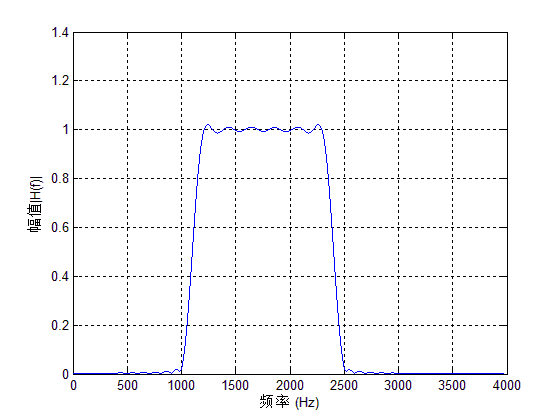窗函数设计法：
根据前面几节的分析：设计一个FIR低通滤波器通常按照下面的步骤执行：

根据滤波器设计要求，确定滤波器的过渡带宽和阻带衰减要求，选择合适窗函数的类型并进行估计窗函数的宽度N。
根据所求的理想滤波器求出单位脉冲响应$h_d(n)$
根据求得的h(n)求出其频率响应。
根据频率响应验证是否满足技术指标。
若不满足指标要求，则应调整窗函数类型或者长度，然后重复(1),(2),(3)(4)步，直到满足要求为止。

注意：matlab中数据通常是以列向量形式存储的，所以两个向量相乘必须进行转置。计算滤波器的单位脉冲响应h(n),根据窗函数设计理论$h(n)=h_d(n)*w(n)$,在matlab中用语句hn=hd*wd实现h(n).
窗函数设计法程序设计如下：
function [h]=usefir1(mode,n,fp,fs,window,r,sample)
% mode:模式(1--高通; 2--低通; 3--带通; 4--带阻)
% n:阶数, 加窗的点数为阶数加1
% fp:高通和低通时指示截止频率, 带通和带阻时指示下限频率
% fs:带通和带阻时指示上限频率
% window:加窗(1--矩形窗; 2--三角窗; 3--巴特窗; 4--汉明窗;
%5--汉宁窗; 6--布莱克曼窗; 7--凯泽窗; 8--契比雪夫窗)
% r代表加chebyshev窗的r值和加kaiser窗时的beta值
% sample:采样率
% h:返回设计好的FIR滤波器系数
if window==1 w=boxcar(n+1);
end
if window==2 w=triang(n+1);end
if window==3 w=bartlett(n+1);end
if window==4 w=hamming(n+1);end
if window==5 w=hanning(n+1);end
if window==6 w=blackman(n+1);end
if window==7 w=kaiser(n+1,r);end
if window==8 w=chebwin(n+1,r);
end
wp=2*fp/sample;
ws=2*fs/sample;
if mode==1 h=fir1(n,wp,'high',w);
end
if mode==2 h=fir1(n,wp,'low',w);
end
if mode==3 h=fir1(n,[wp,ws],w);
end
if mode==4 h=fir1(n,[wp,ws],'stop',w);
end
m=0:n;
subplot(131);
plot(m,h);grid on;
title('冲激响应');
axis([0 n 1.1*min(h) 1.1*max(h)]);
ylabel('h(n)');xlabel('n');
freq_response=freqz(h,1);
magnitude=20*log10(abs(freq_response));
m=0:511; f=m*sample/(2*511);
subplot(132);
plot(f,magnitude);grid on;
title('幅频特性');
axis([0 sample/2 1.1*min(magnitude) 1.1*max(magnitude)]);
ylabel('f幅值');xlabel('频率');
phase=angle(freq_response);
subplot(133);plot(f,phase);grid on;
title('相频特性');
axis([0 sample/2 1.1*min(phase) 1.1*max(phase)]);
ylabel('相位');xlabel('频率');


案例分析：假设需要设计一个40阶的带通FIR滤波器，采用汉明窗，采样频率为10kHz,两个截止频率分别为2kHz和3kHz,则需要在Matlab的命令行窗口输入：
h=usefir1(3,60,2000,3000,4,2,10000);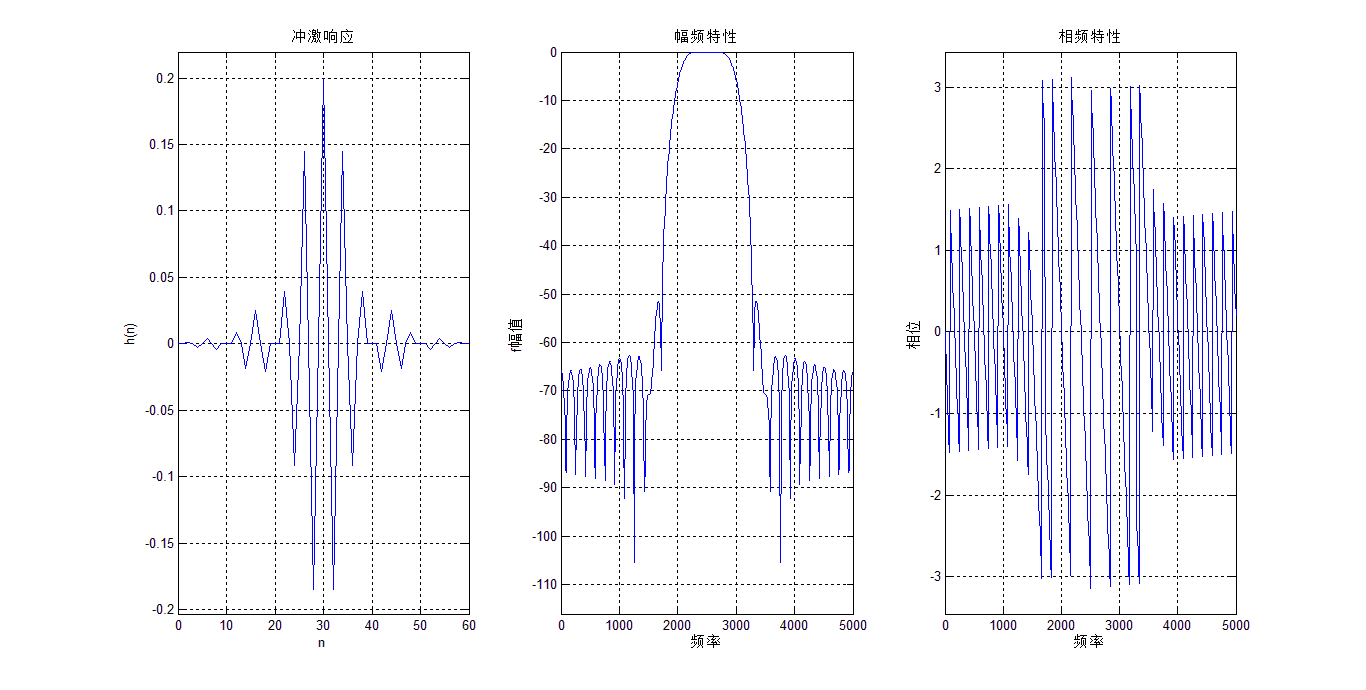展开全文数字信号处理
• 分析了FIR数字滤波器的原理,介绍了采用窗函数法设计FIR数字滤波器的实现过程。Matlab仿真验证了所设计滤波器可以根据需要调整参数,从而实现需要的滤波功能。设计简单、方便,实用性强。
• 1引言 数字滤波是数字信号处理的一种重要算法 广泛用于对信号的...无限脉冲响应IIR 滤波器两类FIR 滤波器的线性与稳定性使其应用更为广泛本文主要介绍采用窗函数法设计FIR 滤波器及其MATLAB 的软件实现方法 2FIR 数字滤
• 数字滤波器有多种设计方法，如双线性变换法、窗函数设计法、插值逼近法和Chebyshev逼近法等等，但是通常采用窗函数法设计窗函数法设计FIR滤波器的基本思想是：根据给定的滤波器技术指标选择滤波器长度N和窗函数ω...
• FIR、IIR数字滤波器是一类重要的滤波器，它用较低的阶数就可以很好地实现频率选择特性，因此在通信、语言与信号处理、HDTV（高画质电视）、...由于窗函数法设计类似只是加的窗函数不同，故本文重点阐述矩形窗的设计方法
• 采用窗函数法、等波纹最佳逼近法设计实现低通、高通、带通、带阻等不同类型的FIR数字滤波器。
• 本文介绍了采用窗函数设计法用Matlab仿真技术和DSP技术来实现FIR滤波器的设计过程，供读者参考学习。
• 数字滤波在研究港内长周期波动中的应用，季小强，冯卫兵， 周期大于20s的长周期波浪一般难以直接观测，需要予以一定的手段对波浪的观测数据进行分离。本文采用窗函数法设计FIR低通数字滤波�
• 采用窗函数设计法，用Matlab代码实现数字滤波器的设计，可选择各种类型的滤波器与各种窗函数相组合。
• 今天给大侠带来FIR数字滤波器设计，由于篇幅较长，分三篇。今天带来第一篇，数字滤波器介绍，包括数字滤波器概述、分类以及设计指标。话不多说，上货。 ...本篇采用窗函数法、频率采样法..
今天给大侠带来FIR数字滤波器设计，由于篇幅较长，分三篇。今天带来第一篇，数字滤波器介绍，包括数字滤波器概述、分类以及设计指标。话不多说，上货。数字滤波器的输入输出均为数字信号，信号通过数字滤波器后，可以改变频率成分的相对比例或滤除某些频率成分。数字滤波器可以分为IIR数字滤波器和FIR数字滤波器。

本篇只介绍FIR数字滤波器的设计，可以根据所给定的频率特性直接设计FIR数字滤波器。FIR数字滤波器在保证幅度特性满足要求的同时，能够做到严格的线性特性。

本篇采用了窗函数法、频率采样法以及基于firls函数和remez函数的最优化方法设计FIR滤波器。对FIR滤波器进行了详细的理论分析，并且对应于每种方法都给出了设计实例。通过编写MATLAB语言程序，运行程序，得到幅频和相频特性图。

对于窗函数和firls函数设计的滤波器，还通过建立Simulink系统模块进行仿真，观察滤波器滤波情况。

数字滤波器

一、数字滤波器的概述

所谓数字滤波器，是指输入输出均为数字信号，通过一定的运算关系，改变输入信号中所含频率成分的相对比例，或滤除某些频率成分的器件。数字滤波器具有稳定性高、精度高、灵活性大等突出优点。对于数字滤波器而言，若系统函数为H(z),其脉冲响应为h(n),输入时间序列为x(n),则它们在时域内的关系式（1-1）如下：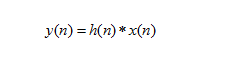在Z域内，输入和输出存在如下关系式（1-2）：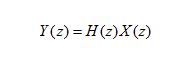上式中， X(z)、Y(z)分别为x(n)和y(n)的Z变换。

在频域内，输入和输出则存在如下关系（1-3）：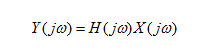上式中，是数字滤波器的频率特性；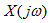和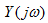分别为x(n)和y(n)的频谱，而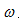为数字角频率。

二、数字滤波器的分类

数字滤波器可以有很多种分类方法，但总体上可分为两大类。一类称为经典滤波器，即一般的滤波器，其特点是输入信号中的有用成分和希望滤除的成分占用不同的频带，通过合适的选频滤波器可以实现滤波。

例如，若输入信号中有干扰，信号和干扰的频带互不重叠，则可滤出信号中的干扰得到纯信号。但是，如果输入信号中信号和干扰的频带相互重叠，则干扰就不能被有效的滤除。

另一类称为现代滤波器，如维纳滤波器、卡尔曼滤波器等，其输入信号中有用信号和希望滤除的频带成分重叠。对于经典滤波器，从频域上也可以分为低通、高通、带通和带阻滤波器。

从时域特性上看，数字滤波器还可以分为有限脉冲响应（FIR，finite impulse response）数字滤波器和无限脉冲响应（IIR, infinite impulse response）数字滤波器。

对于有限脉冲响应（FIR）数字滤波器，其输出y(n)只取决于有限个过去和现在的输入，x(n),x(n-1),…,x(n-m),滤波器的输入输出关系可表示为表达式（1-4），如下：对于无限脉冲响应（IIR）数字滤波器，它的输出不仅取决于过去和现在的输入，而且还取决于过去的输出，其差分方程为表达式（1-5），如下：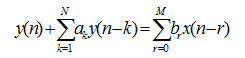该差分方程的单位冲激响应是无限延续的。

三、数字滤波器设计指标

设数字滤波器的传输函数用下式（1-6）表示：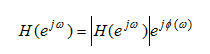式中，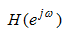为幅频特性，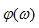为相频特性。幅频特性表示信号通过滤波器后各频率成分的衰减情况，相频特性则反映各频率成分通过滤波器后在时间上的延时情况。通常，选频滤波器的指标要求都以幅频特性给出，对相频特性不作要求，如果需要对输出波形有严格要求，如语音合成、波形传输等，则要求设计线性相位数字滤波器。

数字滤波器的参数指标是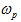、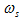、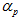和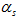。和分别称为通带截止频率和阻带截止频率。通带和阻带内允许的衰减一般用分贝数表示，通带内允许的最大衰减用表示，阻带内允许的最小衰减用表示，和分别定义为关系式（1-7）和关系式（1-8）：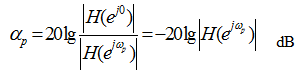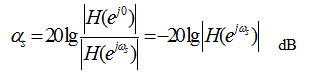式中均假定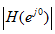已被归一化为1。第一篇就到这里，下一篇带来第二篇，FIR数字滤波器设计基础，包括FIR数字滤波器的特点、线性相位条件以及基本结构。

END

后续会持续更新，带来Vivado、 ISE、Quartus II 、candence等安装相关设计教程，学习资源、项目资源、好文推荐等，希望大侠持续关注。

大侠们，江湖偌大，继续闯荡，愿一切安好，有缘再见！

往期推荐

在FPGA中何时用组合逻辑或时序逻辑

嵌入式中位宽的计算

FPGA Vivado设计流程


展开全文fpga
• 设计FIR滤波器常用的方法有窗函数法与频率抽样法，但是这两种方法均不易精确控制通带与阻带的边界频率，所以在实际应用中有一定的局限性。文中用Matlab语言实现了最佳等波纹FIR滤波器的设计，通过比较显示了它在等...信号调理
• 本文介绍了FIR数字滤波器的结构、特点及设计方法，并采用窗函数法设计FIR滤波器。利用TMS320VC5509 DSP芯片强大的数字信号处理功能实现了该滤波器。实验表明，此数字滤波器工作稳定，能够满足实时的滤波处理功能。信号调理
• 本程序用c语言实现FIR滤波器设计采用的凯撒窗函数法，滤波器阶数、阻带宽度、阻带衰减等都可以通过修改相关系数达到特定的需要，同时通过调整相关系数可以实现带通，带阻，低通，高通等功能。
• 基于IMS320VC5402芯片的数字信号处理功能，采用窗函数法，借助MATLAB程序设计语言，设计FIR数字滤波器，应用DSP汇编语言编程实现了该滤波器。
• ## Matlab 生成fir滤波器抽头系数

万次阅读 多人点赞 2018-12-20 20:09:35
1、 打开 MATLAB 软件，在命令窗口输入 fdatool 并回车，就会弹出滤波器设计工具...其中窗函数设计法在学校课堂中是重点讲解的，提到FIR滤波器肯定会想到hamming、kaiser窗，但是实际应用中却很少使用，因为如果采用...
1、 打开 MATLAB 软件，在命令窗口输入 fdatool 并回车，就会弹出滤波器设计工具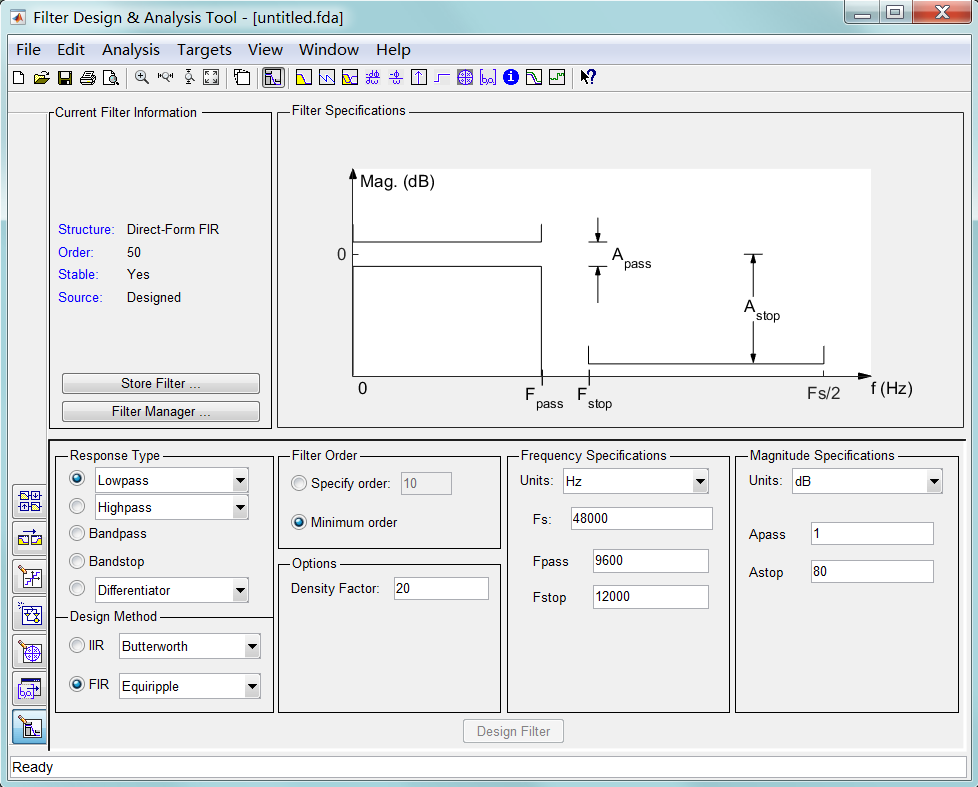2、 FIR滤波器设计方法有多种，，最常用的是窗函数设计法（Window）、等波纹设计法（Equiripple）和最小二乘法 （Least-Squares）等。其中窗函数设计法在学校课堂中是重点讲解的，提到FIR滤波器肯定会想到hamming、kaiser窗，但是实际应用中却很少使用，因为如果采用窗函数设计法，达到所期望的频率响应，与其它方法相比往往阶数会更多；设置频率响应的参数，包括采样频率Fs、通带频率Fpass和阻带频率Fstop

按照实验的要求，在响应类型 Response Type 中选择低通 Lowpass；设计方法 Design Method 中选择 FIR，并且选择用窗函数法 Window 进行 FIR 数字滤波器的设计。在 Filter Order 中选择 Specify order，在这里输入 9，注意这里输入的数值是所要设计的滤波器的阶数减 1。 在 Options 中勾选 Scale Passhand， 并将 Window 选为 Hamming。在 Frequency Specifications 中单位选择 HZ，采样频率 Fs 输入值为 100，截止频率 Fc 中输入值为 10。点击 Design Filter 即可设计出所需的滤波器。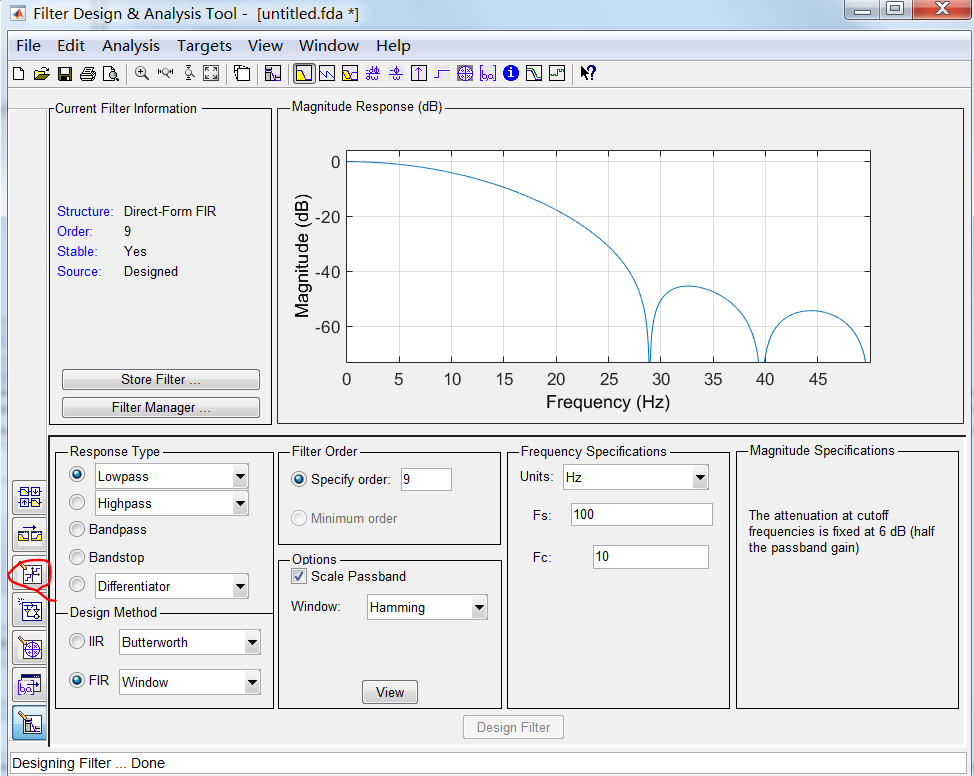3.在 FDATool 工具界面中，点击 File 选择 Export，在弹出窗口中点击 Export，即可在 MATLAB 中生成所设计的滤波器的抽头系数。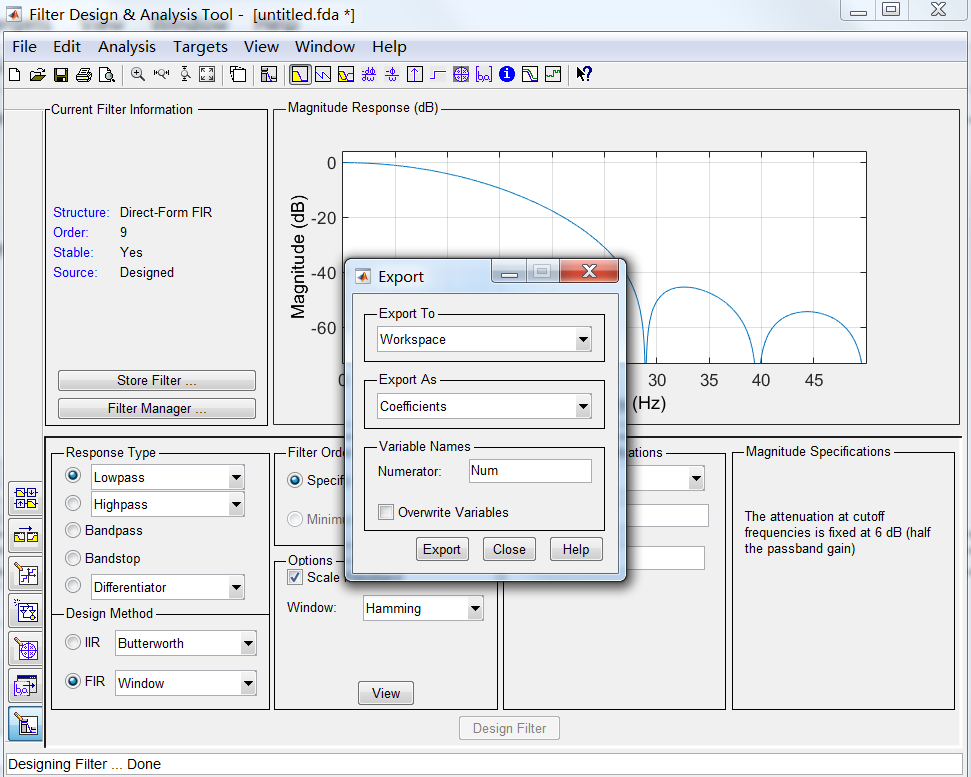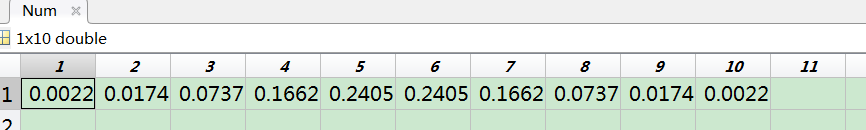这里分出来一小部分空间，引用点别人的内容来简单介绍下上述几个参数的意思：

Response Type：选择FIR滤波器的类型：低通、高通、带通和带阻等。在DDC/DUC模块设计中，抽取和内插需要使用Halfband Lowpass类型，而channel filter需要使用Raised-cosine类型。

Design Method：FIR滤波器设计方法有多种，最常用的是窗函数设计法(Window)、等波纹设计法(Equiripple)和最小二乘法(Least-Squares)等。其中窗函数设计法在学校课堂中是重点讲解的，提到FIR滤波器肯定会想到hamming、kaiser窗，但是实际应用中却很少使用，因为如果采用窗函数设计法，达到所期望的频率响应，与其它方法相比往往阶数会更多；而且窗函数设计法一般只参照通频带wp、抑制频带ws和理想增益来设计滤波器，但是实际应用中通频带和抑制带的波纹也是需要考虑的，那在这种情况下，采用等波纹设计法就非常适用了。

Filter Order：设置滤波器的阶数，这个选项直接影响滤波器的性能，阶数越高，性能越好，但是相应在FPGA实现耗用的资源需要增多。在这个设置中提供2个选项：Specify order和Minimum order，Specify order是工程师自己确定滤波器的阶数，Minimum order是让工具自动确定达到期望的频率相应所需要的最小阶数，因此具体选择哪个选项得视实际情况而定了。

density factor：这个参数控制了频率网的密度。提高这个参数的值可以使设计出的滤波器更加接近理想的频率响应，但这样会增加滤波的计算量。因为滤波器设计要求频率网上每个频点都要满足理想滤波器的指标规格，频率网越密，设计出的滤波器公式越复杂。

Frequency Specification：设置频率响应的参数，包括采样频率Fs、通带频率Fpass和阻带频率Fstop。

magnitude specifications：定义幅值衰减，单位是db，分贝。Apass表示通带衰减，Astop表示阻带衰减。Apass/Astop = 20*log10(输出/输入)。

用等波纹最佳逼近法设计的滤波器，其通带和阻带均为等波纹特性，且通带最大衰减和阻带最小衰减可以分别控制，所以其指标均匀分布，没有资源浪费，所以阶数低得多。http://www.elecfans.com/d/700098.html

4.因为 FPGA 并不支持浮点数的运算，所以需要对抽头系数进行量化处理。在 FDATool 界面中，首先点击按钮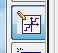，在 Filter arithmetic 中选择 Fixed-point， Number word length 中可以输入的是字长，当输入 8 时，点击 Apply，可以看到有较大的偏差。所以将数值改为 16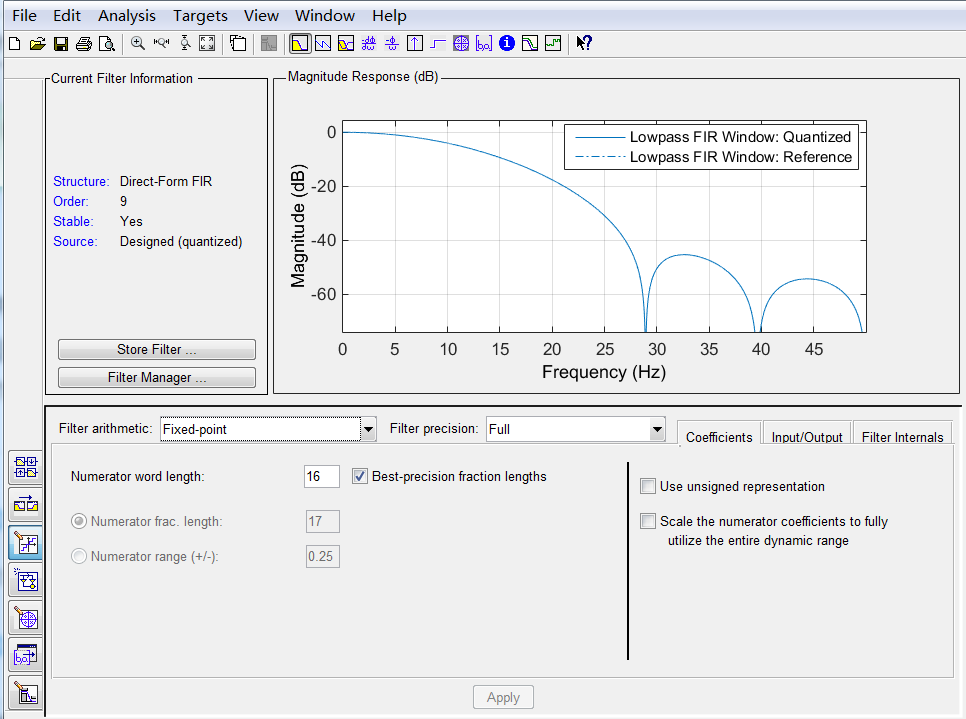5.7、其次，点击 Target，在下拉菜单中选择 XILINX coefficient(.COE) file 即可生成。
以.COE 文件形式保存的经过量化的滤波器系数，当滤波器的阶数较高时可以在 vivado 中
通过 ROM 的 IP 核读入，本次实验的滤波器阶数比较少，所以可以直接将结果复制到滤波器
的 verilog 程序中。

展开全文matlab tap xilinx
• 摘 要： 提出了一种采用现场可编程门阵列器件FPGA并利用窗函数法实现线性FIR数字滤波器硬件电路的方案，并以一个十六阶低通FIR数字滤波器电路的实现为例说明了利用Xilinx公司XC4000系列芯片的设计过程。设计的电路...
• 提出了一种采用现场可编码门阵列器件（FPGA）并利用窗函数法实现线性FIR数字滤波器的设计方案，并以一个十六阶低通FIR数字滤波器电路的实现为例说明了利用Xilinx公司的Virtex-E系列芯片的设计过程。对于在FPGA中实现...
• 摘要：提出了一种采用现场可编码门阵列器件（FPGA）并利用窗函数法实现线性FIR数字滤波器的设计方案，并以一个十六阶低通FIR数字滤波器电路的实现为例说明了利用Xilinx公司的Virtex-E系列芯片的设计过程。...
• 针对数字信号处理及DSP技术教学中的困难，设计1个FIR数字滤波的综合实验。采用窗函数法，借助Matlab程序设计语言，设计FIR数字滤LabVIEW
• 数十个各种详细的波形图片 方便设计采用 语音信号的采集 语音信号的频谱分析 设计数字滤波器和画出频率响应 首先用窗函数法设计高通低通带通三种滤波器，可以利用函数fir1设计FIR滤波器，然后在用双线性变换法设计...
• 在有限脉冲响应（FIR）数字滤波器设计中，讨论了FIR线性相位滤波器的特点和用窗函数法设计FIR滤波器两个问题。两类滤波器整个设计过程都是按照理论分析、编程设计、具体实现的步骤进行的。用Matlab对几种滤波器进行...
• 　Command输入fdatool，例如FIR采用窗函数法设计16阶低通filter，fc（frequency cutoff） = 10800Hz，fs = 48000Hz，输入参数： 需要注意的是由于存在常数项，N阶Filter用N-1阶设计即可。 设计完滤波器并不是...
• 采用凯塞设计一个合适的理想带通滤波器，来实现对音乐信号的滤波去噪。设计时，使用窗口函数设计在MATLAB环境中设计FIR滤波器。
• 摘 要 本文研究了IIR数字滤波器和FIR数字滤波器在...数字滤波器转换在有限脉冲响应FIR数字滤波器设计中研究了FIR线性相位滤波器的特点和用窗函数法设计FIR滤波器两个问题这两类滤波器全部设计过程都是由理论分析编程
• 提出了一种基于FPGA(现场可编程门阵列)采用DDS(直接数字频率合成器)技术的任 意波形发生器设计方案。该方案的硬件电路以FPGA为...VHDL语言设计数控振荡器实现，低通滤波器为采用窗函数法设计的16阶线性FIR数字滤波器。